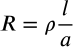Resistance formula
in Formulas
recategorized

0 like 0 dislike

Resistance formula0 like 0 dislike

The formula for resistance is,Where,

R = resistance in Ohms

ρ = resistivity in ohm - metre

l = length

A = cross sectional area